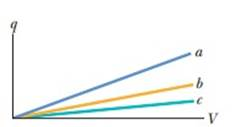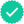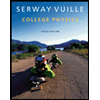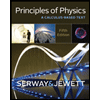#### Concept explainers#### Videos

Textbook QuestionChapter 25, Problem 1Q

Figure 25-18 shows plots of charge versus potential difference for three parallel-plate capacitors that have the plate areas and separations given in the table. Which plot goes with which capacitor?Figure 25-18 Question 1.

 Capacitor Area Separation 1 A d 2 2A d 3 A 2dTo determine

To find:

Which plot goes with which capacitor.

Solution:

Plot a goes with the capacitor 2. The plot b goes with capacitor 1 and plot c goes with capacitor 3.

### Explanation of Solution

1) Concept:

Using Eq.25-9, we can find capacitance of each capacitor from the given values of area and plate separation. Then using Eq.25-1, we can compare the capacitance from the slopes of the plots. Then, comparing the predictions about the capacitance yielded from both the equations, we can find out which plot goes with which capacitor.

2) Formulae:

i) From Eq.25-1, the charge q is

q=CV

ii) From Eq.25-9, the capacitance is

3) Given:

i) For capacitor 1, area is A

ii) For capacitor 2, area is 2A

iii) For capacitor 3, area is A

iv) For capacitor 1, separation is d

v) For capacitor 2, separation is d

vi) For capacitor 3, separation is 2d

4) Calculations:

From Eq.25-1, the charge q is

q=CV

where C is the capacitance and V is the potential difference.

Therefore, the capacitance is given by

C=qV=slope.(1)

From Eq.25-9, the capacitance is

where ε0 is the permittivity, A is the area of the plates and d is the separation between the plates.

Let, A=1 and d=1. Putting it in Eq.25-9 we get,

Capacitor 1 has capacitance,

C1=ε011=ε0

Similarly, Capacitor 2 has capacitance,

C2=ε021=2ε0

And Capacitor 3 has capacitance,

C3=ε012=12ε0

From this, we can interpret that capacitance of capacitor 2 has the greatest value and capacitor 1 has the greater capacitance than that of capacitor 3.

From the graph, we can infer that the plot a has the greatest slope. Hence, the capacitance and the slope of plot b is greater than that of plot c. Hence, corresponding capacitance.

Therefore, we can conclude that, Plot a goes with capacitor 2, plot b goes with capacitor 1 and plot c goes with capacitor 3.

Conclusion:

We can predict about the plots between the charge and the voltage corresponding to capacitors having some area and separation between the plates of capacitor from the slope of the graph and the formula for capacitance.

### Want to see more full solutions like this?

Subscribe now to access step-by-step solutions to millions of textbook problems written by subject matter experts!
03:20
Knowledge BoosterRecommended textbooks for you
•College Physics
Physics
ISBN:9781285737027
Author:Raymond A. Serway, Chris Vuille
Publisher:Cengage LearningPhysics for Scientists and Engineers: Foundations...
Physics
ISBN:9781133939146
Author:Katz, Debora M.
Publisher:Cengage LearningPrinciples of Physics: A Calculus-Based Text
Physics
ISBN:9781133104261
Author:Raymond A. Serway, John W. Jewett
Publisher:Cengage Learning
•Physics for Scientists and Engineers with Modern ...
Physics
ISBN:9781337553292
Author:Raymond A. Serway, John W. Jewett
Publisher:Cengage LearningPhysics for Scientists and Engineers
Physics
ISBN:9781337553278
Author:Raymond A. Serway, John W. Jewett
Publisher:Cengage LearningCollege Physics
Physics
ISBN:9781305952300
Author:Raymond A. Serway, Chris Vuille
Publisher:Cengage Learning
•College Physics
Physics
ISBN:9781285737027
Author:Raymond A. Serway, Chris Vuille
Publisher:Cengage LearningPhysics for Scientists and Engineers: Foundations...
Physics
ISBN:9781133939146
Author:Katz, Debora M.
Publisher:Cengage LearningPrinciples of Physics: A Calculus-Based Text
Physics
ISBN:9781133104261
Author:Raymond A. Serway, John W. Jewett
Publisher:Cengage LearningPhysics for Scientists and Engineers with Modern ...
Physics
ISBN:9781337553292
Author:Raymond A. Serway, John W. Jewett
Publisher:Cengage LearningPhysics for Scientists and Engineers
Physics
ISBN:9781337553278
Author:Raymond A. Serway, John W. Jewett
Publisher:Cengage LearningCollege Physics
Physics
ISBN:9781305952300
Author:Raymond A. Serway, Chris Vuille
Publisher:Cengage Learning